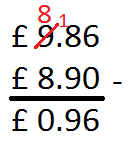# Subtract Money Using Column Method

In this worksheet, students will subtract sums of money (in pounds and pence) using column subtraction with borrowing.Key stage:  KS 2

Curriculum topic:   Number: Addition and Subtraction

Curriculum subtopic:   Add/Subtract up to Four-Digit Numbers

Popular topics:   Money worksheets

Difficulty level:#### Worksheet Overview

In this activity, we will be subtracting sums of money using column subtraction with borrowing.

Example

Subtract the following:

£ 9.86 - £ 8.90

We set out a subtraction in columns, lining up the decimal points carefully.

We do the calculation in the usual way, remembering to borrow if necessary.Note:  Remember, we can't take 9 away from 8, so we have to 'borrow' from the next column.  The 8 (80) becomes 18 (180)

Remember, there might be more than one way to write a correct answer when you calculate with money.

£0.96 = 96 penceLet's have a go at some questions now.

### What is EdPlace?

We're your National Curriculum aligned online education content provider helping each child succeed in English, maths and science from year 1 to GCSE. With an EdPlace account you’ll be able to track and measure progress, helping each child achieve their best. We build confidence and attainment by personalising each child’s learning at a level that suits them.

Get started